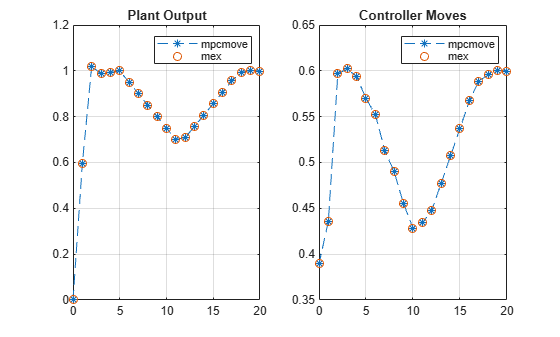Main Content

# Generate Code to Compute Optimal MPC Moves in MATLAB

This example shows how to use the `mpcmoveCodeGeneration` command to generate C code to compute optimal MPC control moves for real-time applications.

### Plant Model

The plant is a single-input, single-output, stable, 2nd order linear plant.

`plant = tf(5,[1 0.8 3]);`

Convert the plant to discrete-time, state-space form, and specify a zero initial states vector.

```Ts = 1; plant = ss(c2d(plant,Ts)); x0 = zeros(size(plant.B,1),1);```

### Design MPC Controller

Create an MPC controller with default horizons.

`mpcobj = mpc(plant,Ts);`
```-->The "PredictionHorizon" property of "mpc" object is empty. Trying PredictionHorizon = 10. -->The "ControlHorizon" property of the "mpc" object is empty. Assuming 2. -->The "Weights.ManipulatedVariables" property of "mpc" object is empty. Assuming default 0.00000. -->The "Weights.ManipulatedVariablesRate" property of "mpc" object is empty. Assuming default 0.10000. -->The "Weights.OutputVariables" property of "mpc" object is empty. Assuming default 1.00000. ```

Specify controller tuning weights.

```mpcobj.Weights.MV = 0; mpcobj.Weights.MVrate = 0.5; mpcobj.Weights.OV = 1;```

Specify initial constraints on the manipulated variable and plant output. These constraints will be updated at run time.

```mpcobj.MV.Min = -1; mpcobj.MV.Max = 1; mpcobj.OV.Min = -1; mpcobj.OV.Max = 1;```

### Simulate Online Constraint Changes with `mpcmove` Command

In the closed-loop simulation, constraints are updated and fed into the `mpcmove` command at each control interval.

```yMPCMOVE = []; uMPCMOVE = [];```

Set the simulation time.

`Tsim = 20;`

Initialize the online constraint data.

```MVMinData = -0.2-[1 0.95 0.9 0.85 0.8 0.75 0.7 0.65 0.6 0.55 0.5 ... 0.55 0.6 0.65 0.7 0.75 0.8 0.85 0.9 0.95 1]; MVMaxData = 0.2+[1 0.95 0.9 0.85 0.8 0.75 0.7 0.65 0.6 0.55 0.5 ... 0.55 0.6 0.65 0.7 0.75 0.8 0.85 0.9 0.95 1]; OVMinData = -0.2-[1 0.95 0.9 0.85 0.8 0.75 0.7 0.65 0.6 0.55 0.5 ... 0.55 0.6 0.65 0.7 0.75 0.8 0.85 0.9 0.95 1]; OVMaxData = 0.2+[1 0.95 0.9 0.85 0.8 0.75 0.7 0.65 0.6 0.55 0.5 ... 0.55 0.6 0.65 0.7 0.75 0.8 0.85 0.9 0.95 1];```

Initialize plant states.

`x = x0;`

Initialize MPC states.

`xmpc = mpcstate(mpcobj);`
```-->Assuming output disturbance added to measured output channel #1 is integrated white noise. -->The "Model.Noise" property of the "mpc" object is empty. Assuming white noise on each measured output channel. ```

Run a closed-loop simulation by calling `mpcmove` in a loop.

```options = mpcmoveopt; for ct = 1:round(Tsim/Ts)+1 % Update and store plant output. y = plant.C*x; yMPCMOVE = [yMPCMOVE y]; % Update constraints. options.MVMin = MVMinData(ct); options.MVMax = MVMaxData(ct); options.OutputMin = OVMinData(ct); options.OutputMax = OVMaxData(ct); % Compute control actions. u = mpcmove(mpcobj,xmpc,y,1,[],options); % Update and store plant state. x = plant.A*x + plant.B*u; uMPCMOVE = [uMPCMOVE u]; end```

### Validate Simulation Results with `mpcmoveCodeGeneration` Command

To prepare for generating code that computes optimal control moves from MATLAB, it is recommended to reproduce the same control results with the `mpcmoveCodeGeneration` command before using the `codegen` command from the MATLAB Coder product.

```yCodeGen = []; uCodeGen = [];```

Initialize plant states.

`x = x0;`

Create data structures to use with `mpcmoveCodeGeneration using` `getCodeGenerationData`.

`[coredata,statedata,onlinedata] = getCodeGenerationData(mpcobj);`

Run a closed-loop simulation by calling `mpcmoveCodeGeneration` in a loop.

```for ct = 1:round(Tsim/Ts)+1 % Update and store plant output. y = plant.C*x; yCodeGen = [yCodeGen y]; % Update measured output in online data. onlinedata.signals.ym = y; % Update reference in online data. onlinedata.signals.ref = 1; % Update constraints in online data. onlinedata.limits.umin = MVMinData(ct); onlinedata.limits.umax = MVMaxData(ct); onlinedata.limits.ymin = OVMinData(ct); onlinedata.limits.ymax = OVMaxData(ct); % Compute control actions. [u,statedata] = mpcmoveCodeGeneration(coredata,statedata,onlinedata); % Update and store plant state. x = plant.A*x + plant.B*u; uCodeGen = [uCodeGen u]; end```

The simulation results are identical to those using `mpcmove`.

```t = 0:Ts:Tsim; figure; subplot(1,2,1) plot(t,yMPCMOVE,'--*',t,yCodeGen,'o'); grid legend('mpcmove','codegen') title('Plant Output') subplot(1,2,2) plot(t,uMPCMOVE,'--*',t,uCodeGen,'o'); grid legend('mpcmove','codegen') title('Controller Moves')```### Generate MEX Function From `mpcmoveCodeGeneration` Command

To generate C code from the `mpcmoveCodeGeneration` command, use the `codegen` command from the MATLAB Coder product. In this example, generate a MEX function `mpcmoveMEX` to reproduce the simulation results in MATLAB. You can change the code generation target to C/C++ static library, dynamic library, executable, etc. by using a different set of `coder.config` settings.

When generating C code for the `mpcmoveCodeGeneration` command:

• Since no data integrity checks are performed on the input arguments, you must make sure that all the input data has the correct types, dimensions, and values.

• You must define the first input argument, `mpcmove_struct`, as a constant when using the `codegen` command.

• The second input argument, `mpcmove_state`, is updated by the command and returned as the second output. In most cases, you do not need to modify its contents and should simply pass it back to the command in the next control interval. The only exception is when custom state estimation is enabled, in which case you must provide the current state estimation using this argument.

```if ~license ('test', 'MATLAB_Coder') disp('MATLAB Coder(TM) is required to run this example.') return end```

Generate MEX function.

```fun = 'mpcmoveCodeGeneration'; funOutput = 'mpcmoveMEX'; Cfg = coder.config('mex'); Cfg.DynamicMemoryAllocation = 'off'; codegen('-config',Cfg,fun,'-o',funOutput,'-args',... {coder.Constant(coredata),statedata,onlinedata});```
```Code generation successful. ```

Initialize data storage.

```yMEX = []; uMEX = [];```

Initialize plant states.

`x = x0;`

Use `getCodeGenerationData` to create data structures to use with `mpcmoveCodeGeneration`.

`[coredata,statedata,onlinedata] = getCodeGenerationData(mpcobj);`

Run a closed-loop simulation by calling the generated `mpcmoveMEX` functions in a loop.

```for ct = 1:round(Tsim/Ts)+1 % Update and store the plant output. y = plant.C*x; yMEX = [yMEX y]; % Update measured output in online data. onlinedata.signals.ym = y; % Update reference in online data. onlinedata.signals.ref = 1; % Update constraints in online data. onlinedata.limits.umin = MVMinData(ct); onlinedata.limits.umax = MVMaxData(ct); onlinedata.limits.ymin = OVMinData(ct); onlinedata.limits.ymax = OVMaxData(ct); % Compute control actions. [u,statedata] = mpcmoveMEX(coredata,statedata,onlinedata); % Update and store the plant state. x = plant.A*x + plant.B*u; uMEX = [uMEX u]; end```

The simulation results are identical to those using `mpcmove`.

```figure subplot(1,2,1) plot(t,yMPCMOVE,'--*',t,yMEX,'o') grid legend('mpcmove','mex') title('Plant Output') subplot(1,2,2) plot(t,uMPCMOVE,'--*',t,uMEX,'o') grid legend('mpcmove','mex') title('Controller Moves')```## Support

#### Implementing an Adaptive Cruise Controller with Simulink

Download technical paper